Data Structures and Algorithms with Object-Oriented Design Patterns in C### Applications

The applications of lists and ordered lists are myriad. In this section we will consider only one--the use of an ordered list to represent a polynomial. In general, an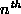-order polynomial in x, for non-negative integer n, has the form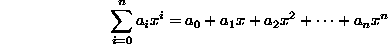where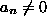. The term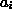is the coefficient of the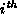power of x. We shall assume that the coefficients are real numbers.

An alternative representation for such a polynomial consists of a sequence of ordered pairs: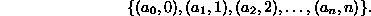Each ordered pair,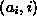, corresponds to the term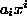of the polynomial. That is, the ordered pair is comprised of the coefficient of theterm together with the subscript of that term, i. For example, the polynomial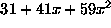can be represented by the sequence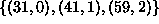.

Consider now the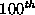-order polynomial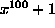. Clearly, there are only two nonzero coefficients: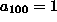and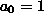. The advantage of using the sequence of ordered pairs to represent such a polynomial is that we can omit from the sequence those pairs that have a zero coefficient. We represent the polynomialby the sequence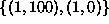Now that we have a way to represent polynomials, we can consider various operations on them. For example, consider the polynomial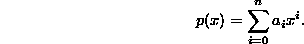We can compute its derivative  with respect to x by differentiating  each of the terms to get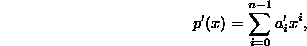where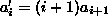. In terms of the corresponding sequences, if p(x) is represented by the sequence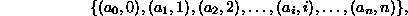then its derivative is the sequence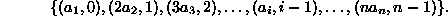This result suggests a very simple algorithm to differentiate a polynomial which is represented by a sequence of ordered pairs:

1. Drop the ordered pair that has a zero exponent.
2. For every other ordered pair, multiply the coefficient by the exponent, and then subtract one from the exponent.
Since the representation of an-order polynomial has at most n+1 ordered pairs, and since a constant amount of work is necessary for each ordered pair, this is inherently an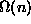algorithm.

Of course, the worst-case running time of the polynomial differentiation will depend on the way that the sequence of ordered pairs is implemented. We will now consider an implementation that makes use of the OrderedListAsLinkedList class. To begin with, we need a class to represent the terms of the polynomial. Programgives the definition of the Term class and several of its methods.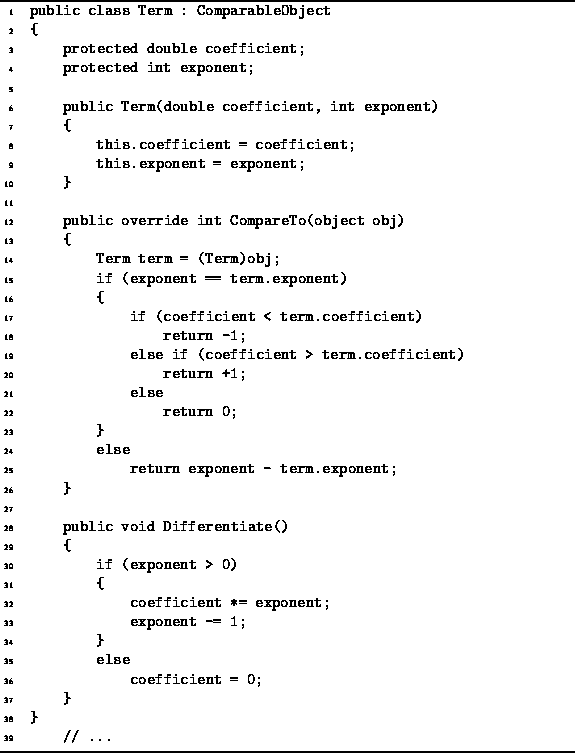Program: Term class.

Each Term instance has two fields, coefficient and exponent, which correspond to the elements of the ordered pair as discussed above. The former is a double and the latter, an int.

The Term class extends the ComparableObject class introduced in Program. Therefore, instances of the Term class may be put into a container. Programdefines three methods: a constructor, CompareTo, and Differentiate. The constructor simply takes a pair of arguments and initializes the corresponding fields accordingly.

The CompareTo method is used to compare two Term instances. Consider two terms ,and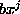. We define the relation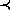on terms of a polynomial as follows: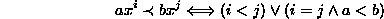Note that the relationdoes not depend on the value of the variable x. The CompareTo method implements therelation.

Finally, the Differentiate method does what its name says: It differentiates a term with respect to x. Given a term such as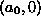, it computes the result (0,0); and given a term such aswhere i>0, it computes the result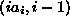.

We now consider the representation of a polynomial. Programdefines the Polynomial abstract class. The class comprises three abstract methods--Add, Differentiate, and Plus. The Add method is used to add terms to a polynomial. The Differentiate method differentiates the polynomial. The Plus method is used to compute the sum of two polynomials. In addition to these methods, the addition operator + is overloaded for Polynomial operands.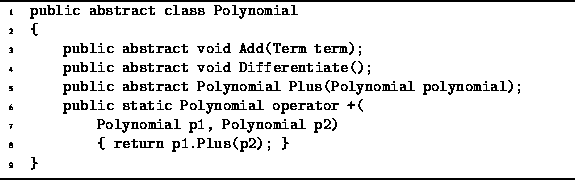Program: Polynomial abstract class.

Programintroduces the PolynomialAsOrderedList class. This concrete class implements the Polynomial interface. It has a single field of type OrderedList. In this case, an instance of the OrderedListAsLinkedList class is used to contain the terms of the polynomial.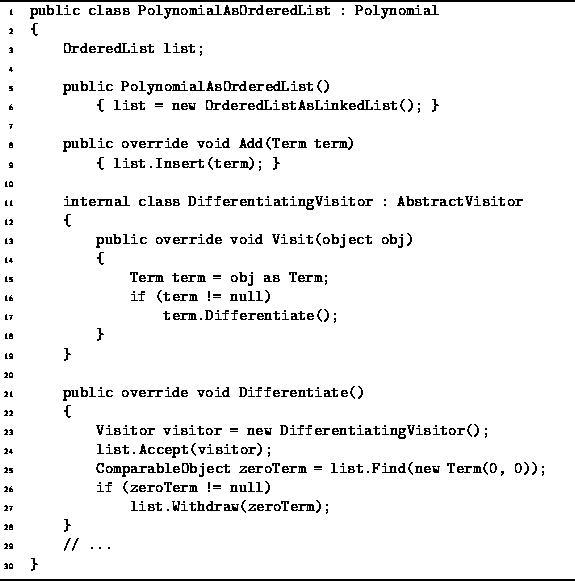Program: PolynomialAsOrderedList class.

Programdefines the method Differentiate which has the effect of changing the polynomial to its derivative with respect to x. To compute this derivative, it is necessary to call the Differentiate method of the Term class for each term in the polynomial. Since the polynomial is implemented as a container, there is an Accept method which can be used to perform a given operation on all of the objects in that container. In this case, we define a visitor, DifferentiatingVisitor, which assumes its argument is an instance of the Term class and differentiates it.

After the terms in the polynomial have been differentiated, it is necessary to check for the term (0,0) which arises from differentiating. The Find method is used to locate the term, and if one is found the Withdraw method is used to remove it.

The analysis of the running time of the polynomial Differentiate method is straightforward. The running time required to differentiate a term is clearly O(1). So too is the running time of the Visit method of the DifferentiatingVisitor. The latter method is called once for each contained object. In the worst case, given an-order polynomial, there are n+1 terms. Therefore, the time required to differentiate the terms is O(n). Locating the zero term is O(n) in the worst case, and so too is deleting it. Therefore, the total running time required to differentiate a-order polynomial is O(n).Copyright © 2001 by Bruno R. Preiss, P.Eng. All rights reserved.